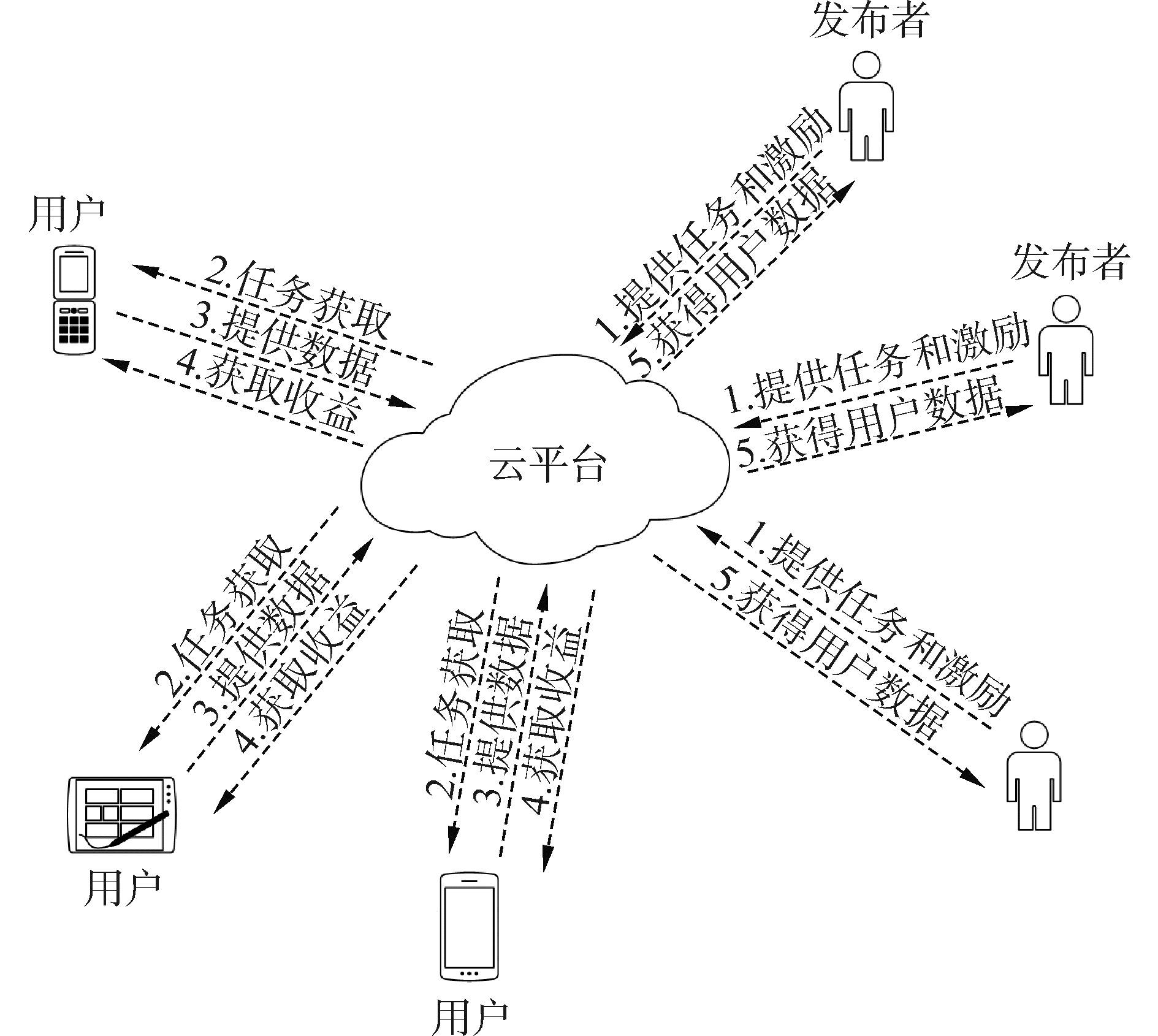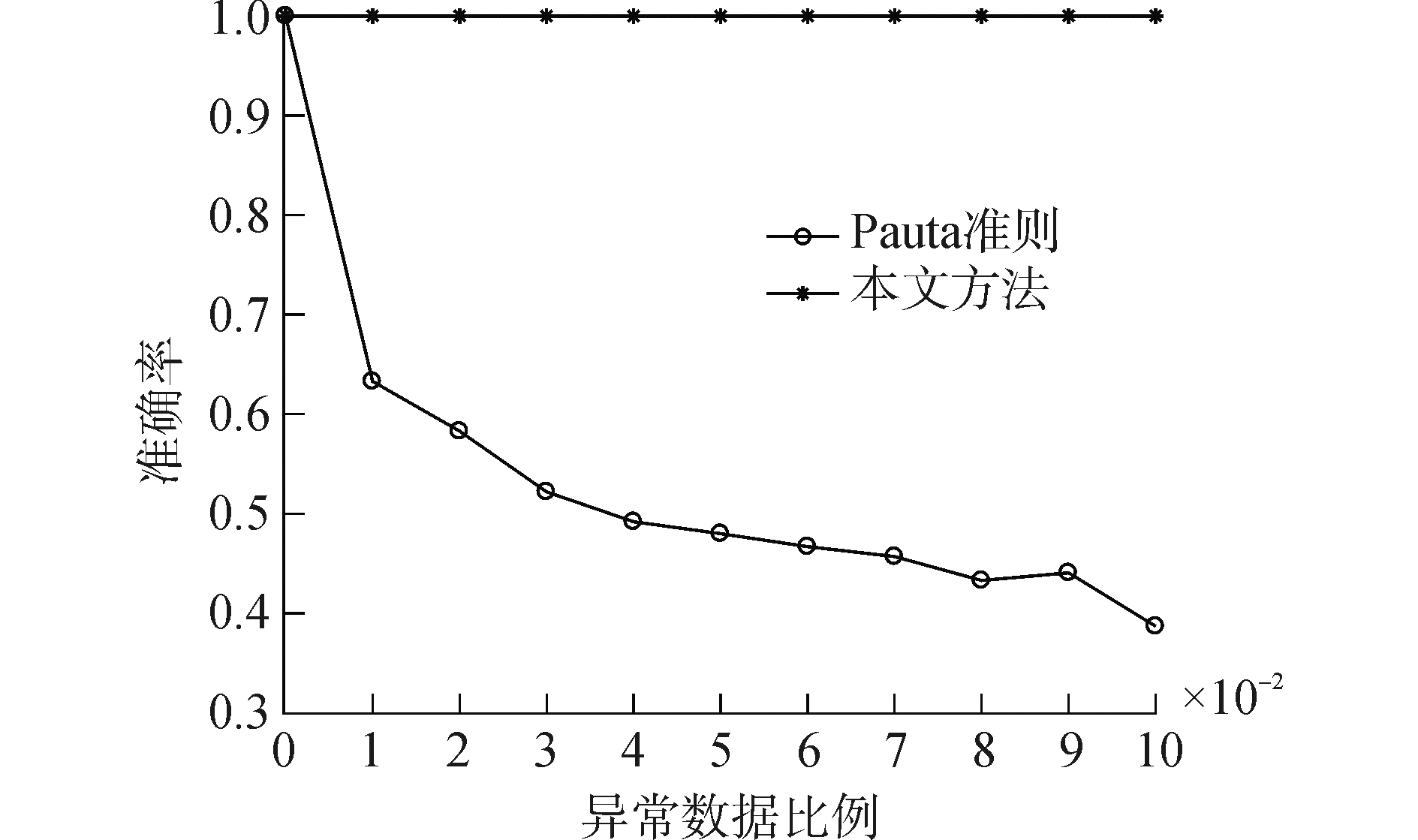﻿ 基于消错决策理论的数据质量评估方法
«上一篇文章快速检索 高级检索

 哈尔滨工程大学学报2018, Vol. 39Issue (12): 2040-2045  DOI: 10.11990/jheu.2018080030

### 引用本文CHEN Yu, LIU Yuanan, SHAO Zihao. Data quality evaluation method based on the error-eliminating decision-making theory[J]. Journal of Harbin Engineering University, 2018, 39(12), 2040-2045. DOI: 10.11990/jheu.201808003.### 文章历史

1. 北京邮电大学 电子工程学院, 北京 100876;
2. 哈尔滨工程大学 计算机科学与技术学院, 黑龙江 哈尔滨 150001

Data quality evaluation method based on the error-eliminating decision-making theory
CHEN Yu 1, LIU Yuanan 1, SHAO Zihao 2
1. School of Electronic Engineering, Beijing University of Posts and Telecommunications, Beijing 100876, China;
2. College of Computer Science and Technology, Harbin Engineering University, Harbin 150001, China
Abstract: In mobile crowd-sensing scenarios, it is difficult to guarantee and evaluate the quality of data collected from a number of mobile users. To solve this problem, we proposed a novel technique for evaluating the quality of crowd-sensing data based on the error-eliminating decision-making theory with regard to error avoidance as the objective. First, we evaluated the quality of data collected from the mobile users by introducing the error-eliminating decision-making theory, wherein low-quality and abnormal data can be identified. Then, in consideration with various requirements regarding data quality for different sensing tasks, we introduced a weight factor for sequencing and evaluating data quality. Our experimental results show that the proposed method can accurately identify low-quality and abnormal data as well as achieve the on-demand sequencing of data quality with respect to different task requirements. In terms of identifying low-quality and abnormal data, our proposed method demonstrates higher recognition accuracy than the Laidda criterion. Additionally, as compared with the ideal-point and TOPSIS methods, our proposed method exhibits high accuracy and simplicity, proving to be advantageous in terms of data quality ranking.
Keywords: mobile crowd-sensing    error-eliminating decision-making theory    data quality evaluation    abnormal data

1 移动群智感知模型 1.1 群智感知的基本思想

“群智感知”的概念雏形可追溯到美国《连线》的著名记者J. Surowiecki的《The Wisdom of Crowds》一书，其中明确指出了群体感知/决策优于个体感知/决策这一普遍性原理，这一思想因而又被称为群体智慧“Crowd Wisdom”或者集体智慧“Collective Intelligence”。群智感知的思想被提出以后，先后经历了众包计算“Crowd sourcing Computing”，机会感知“Opportunistic Sensing”，并随着智能终端的普及而演化为今天的移动群智感知“MCS”。

1.2 移动群智感知系统模型Download: 图 1 移动群智感知系统模型 Fig. 1 System model of mobile crowdsensing

2 数据质量评估方法

2.1 数据识别

 $\begin{array}{l} {t_{i, j}} = \left\{ \begin{array}{l} \rho , \;\;\;\;\;\;\;\;\;\;\;\;\;\;\;\;\;\;\;{g_{i, j}} > z_j^{\max }{\rm{或}}{g_{i, j}} < z_j^{\min }\\ \frac{{{g_{i, j}} - z_j^{\min }}}{{z_j^{\max } - z_j^{\min }}}, \;\;\;\;\;\;\;z_j^{\min } \le {g_{i, j}} \le z_j^{\max } \end{array} \right.\\ \;\;\;\;\;\;\;\;\;\;i = 1, 2, \cdots , m, j = 1, 2, \cdots , n。\end{array}$ (1)

 $\begin{array}{l} {t_{i, j}} = \left\{ \begin{array}{l} \rho , \;\;\;\;\;\;\;\;\;\;\;\;\;\;\;\;\;\;\;{g_{i, j}} < z_j^{\min }{\rm{或}}{g_{i, j}} > z_j^{\max }\\ \frac{{z_j^{\max } - {g_{i, j}}}}{{z_j^{\max } - z_j^{\min }}}, \;\;\;\;\;\;\;z_j^{\min } \le {g_{i, j}} \le z_j^{\max } \end{array} \right.\\ \;\;\;\;\;\;\;\;\;\;i = 1, 2, \cdots , m, j = 1, 2, \cdots , n。\end{array}$ (2)

 $t_i^{\max } = \max \left\{ {{t_{i, j}}} \right\}$ (3)

timax=ρ时，该用户数据不满足条件，即为错误数据，去除该数据。通过以上方法可以实现对用户数据质量的识别，实现了对低质量与异常数据的处理。

2.2 数据排序

 $\left\{ \begin{array}{l} Z_j^{\max } = \frac{{z_j^{\max }}}{{z_j^{\max }}}{\rm{ = }}1\\ Z_j^{\min } = \frac{{z_j^{\min }}}{{z_j^{\max }}} \end{array} \right.$ (4)

 $E_j^* = \frac{{{{\left( {Z_j^{\max } - Z_j^{\min }} \right)}^2}}}{{\sum\limits_{i = 1}^n {{{\left( {Z_i^{\max } - Z_i^{\min }} \right)}^2}} }}$ (5)

 $\begin{array}{l} \left\{ \begin{array}{l} {r_{u!}} = \left\{ {{\omega _1}{t_{1, 1}}E_1^*, {\omega _2}{t_{1, 2}}E_2^*, \cdots , {\omega _j}{t_{1, j}}E_j^*} \right\}\\ {r_{{u_2}}} = \left\{ {{\omega _1}{t_{2, 1}}E_1^*, {\omega _2}{t_{2, 2}}E_2^*, \cdots , {\omega _j}{t_{2, j}}E_j^*} \right\}\\ \cdots \cdots \\ {r_{{u_i}}} = \left\{ {{\omega _1}{t_{i, 1}}E_1^*, {\omega _2}{t_{i, 2}}E_2^*, \cdots , {\omega _j}{t_{i, j}}E_j^*} \right\} \end{array} \right.\\ i = 1, 2, \cdots , m, j = 1, 2, \cdots , n, \sum\limits_{j = 1}^n {{\omega _j} = 1。} \end{array}$ (6)

 ${R_i} = \sqrt {\sum\limits_{j = 1}^n {{{\left( {{\omega _j}{t_{i, j}}E_j^*} \right)}^2}} } , \;\;\;\;i = 1, 2, \cdots , m$ (7)
2.3 算法描述

1) 初始化

2) 确定用户数据质量评价指标cj

3) for i=1, 2, …, m, j=1, 2, …, n do

4) 计算gi, j

5) 判断质量评价指标cj

6) if cjCcost

7) 通过式(1)计算用户数据的错误值timax

8) else

9) 通过式(2)计算用户数据的错误值timax

10) end if

11) 通过式(3)计算用户数据最大错误值timax

12) if timax=ρ

13) 通过式(5)计算数据质量评价指标极限损失值Ej*

14) 通过式(6)计算用户数据错误损失序列rui

15) 对用户数据进行排序

16) else

17) 剔除该用户数据

18) end for

3 实验结果与分析 3.1 实验设置表 1 指标量化 Table 1 Index quantification

3.2 数据识别对比

 ${X_{dr}} = \frac{{{\rm{su}}{{\rm{m}}_{tr}}}}{{{\rm{su}}{{\rm{m}}_{ar}}}}$ (8)Download: 图 2 准确率对比 Fig. 2 Accuracy comparison

3.3 数据排序对比表 2 用户数据 Table 2 User data

1) 时间优选。该策略更关注用户的响应时间和任务距离，适用于紧急情形下的数据质量评估。因此在时间优选策略中，指标1和指标2将获得较大权重。实验中采取的权重参数为：ω1=0.35，ω2=0.35，ω3=0.15，ω4=0.15。

2) 质量优先。该策略更关注用户的收集方式与收集声音所处的环境，通常适用于范围广、数据量需求大情况下的数据质量评估。因此，在质量优选策略中，指标3和指标4将获得较大权重。实验中采取的权重参数为：ω1=0.15，ω2=0.15，ω3=0.35，ω4=0.35。表 3 不同方法对比 Table 3 Comparison of different methods

4 结论

1) 本文针对移动群智感知系统中的数据质量评估问题，从规避错误的角度出发，提出了一种基于消错决策理论的数据质量评估方法，弥补了当前对数据质量评估研究的缺失。

2) 实验结果表明:所提出的方法不仅实现了对低质量和异常数据的识别，还通过权值的引入，实现了移动群智感知中对不同任务需求数据进行按需排序，更符合实际需求。

3) 在对低质量和异常数据识别中，相对于拉依达准则，具有识别准确性高的优点；在对数据质量排序上，相对于理想点法、TOPSIS法，具有排序准确性更高、方法简单高效并可按需分配的优点。

  ZHANG Xinglin, YANG Zheng, SUN Wei, et al. Incentives for mobile crowd sensing:a survey[J]. IEEE communications surveys & tutorials, 2016, 18(1): 54-67. (0)  ALVEAR O, CALAFATE C, CANO J C, et al. Crowdsensing in smart cities:overview, platforms, and environment sensing issues[J]. Sensors, 2018, 18(2): 460. DOI:10.3390/s18020460 (0)  WANG Xiong, ZHANG Jinbei, TIAN Xiaohua, et al. Crowdsensing-based consensus incident report for road traffic acquisition[J]. IEEE transactions on intelligent transportation systems, 2018, 19(8): 2536-2547. DOI:10.1109/TITS.2017.2750169 (0)  GUO Bin, WANG Zhu, YU Zhiwen, et al. Mobile crowd sensing and computing:The review of an emerging human-powered sensing paradigm[J]. ACM computing surveys, 2015, 48(1): 7. (0)  JIANG Changkun, GAO Lin, DUAN Lingjie, et al. Exploiting data reuse in mobile crowdsensing[C]//2016 IEEE Global Communications Conference. Washington, DC, USA, 2017: 1-6. (0)  DU Pengfei, YANG Qinghai, HE Qingsu, et al. Energy-aware quality of information maximisation for wireless sensor networks[J]. IET communications, 2016, 10(17): 2281-2289. DOI:10.1049/iet-com.2015.1064 (0)  吴垚, 曾菊儒, 彭辉, 等. 群智感知激励机制研究综述[J]. 软件学报, 2016, 27(8): 2025-2047. WU Yao, ZENG Juru, PENG Hui, et al. Survey on incentive mechanisms for crowd sensing[J]. Journal of software, 2016, 27(8): 2025-2047. (0)  南文倩, 郭斌, 陈荟慧, 等. 基于跨空间多元交互的群智感知动态激励模型[J]. 计算机学报, 2015, 38(12): 2412-2425. NAN Wenqian, GUO Bin, CHEN Huihui, et al. A cross-space, multi-interaction-based dynamic incentive mechanism for mobile crowd sensing[J]. Chinese journal of computers, 2015, 38(12): 2412-2425. (0)  PENG Dan, WU Fan, CHEN Guihai. Data quality guided incentive mechanism design for crowdsensing[J]. IEEE transactions on mobile computing, 2018, 17(2): 307-319. DOI:10.1109/TMC.2017.2714668 (0)  ZHOU Tongqing, CAI Zhiping, CHEN Yueyue, et al. Improving data credibility for mobile crowdsensing with clustering and logical reasoning[C]//Second International Conference on Cloud Computing and Security. Nanjing, 2016: 138-150. (0)  LIU Shengzhong, ZHENG Zhenzhe, WU Fan, et al. Context-aware data quality estimation in mobile crowdsensing[C]//IEEE INFOCOM 2017-IEEE Conference on Computer Communications. Atlanta, GA, USA, 2017: 1-9. (0)  SUROWIECKI J. The wisdom of crowds:Why the many are smarter than the few and how collective wisdom shapes business, economics, societies and nations[J]. Personnel psychology, 2006, 59(4): 982-985. DOI:10.1111/peps.2006.59.issue-4 (0)  MALONE T W, LAUBACHER R, DELLAROCAS C. Harnessing crowds: mapping the genome of collective intelligence. MIT Sloan Research Paper No. 4732-09[R]. Cambridge, Massachusetts: Massachusetts Institute of Technology, 2009. (0)  DOAN A, RAMAKRISHNAN R, HALEVY A Y. Crowdsourcing systems on the world-wide web[J]. Communications of the ACM, 2011, 54(4): 86-96. DOI:10.1145/1924421 (0)  LANE N D, MILUZZO E, LU Hong, et al. A survey of mobile phone sensing[J]. IEEE communications magazine, 2010, 48(9): 140-150. DOI:10.1109/MCOM.2010.5560598 (0)  HUANG Haoran, CAI Ken. A method of fuzzy multiple attribute decision making based on the error-eliminating theory[J]. Journal of intelligent & fuzzy systems, 2016, 31(4): 2119-2127. (0)  MARJANOVIĆ M, SKORIN-KAPOV L, PRIPUŽIĆ K, et al. Energy-aware and quality-driven sensor management for green mobile crowd sensing[J]. Journal of network and computer applications, 2016, 59: 95-108. DOI:10.1016/j.jnca.2015.06.023 (0)  丁小欧, 王宏志, 张笑影, 等. 数据质量多种性质的关联关系研究[J]. 软件学报, 2016, 27(7): 1626-1644. DING Xiaoou, WANG Hongzhi, ZHANG Xiaoying, et al. Association relationships study of multi-dimensional data quality[J]. Journal of software, 2016, 27(7): 1626-1644. (0)